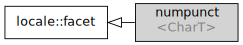/C++

# std::numpunct

Defined in header `<locale>`
```template< class CharT >
class numpunct;```

The facet `std::numpunct` encapsulates numeric punctuation preferences. Stream I/O operations use `std::numpunct` through `std::num_get` and `std::num_put` for parsing numeric input and formatting numeric output.

The numbers that are supported by `std::numpunct` have the format described below. Here `digit` represents the radix set specified by the `fmtflags` argument value, `thousands-sep` and `decimal-point` are the results of `thousands_sep()` and `decimal_point()` functions respectively. The format of integer values is as follows:

```integer     ::= [sign] units
sign        ::= plusminus
plusminus   ::= '+' | '-'
units       ::= digits [thousands-sep units]
digits      ::= digit [digits]```

The number of digits between the `thousand-sep`s (maximum size of `digits`) is specified by the result of `grouping()`.

The format of floating-point values is as follows:

```floatval    ::= [sign] units [decimal-point [digits]] [e [sign] digits] |
[sign]        decimal-point  digits   [e [sign] digits]
e           ::= 'e' | 'E'```Inheritance diagram.

Two standalone (locale-independent) specializations are provided by the standard library:

Defined in header `<locale>`
`std::numpunct<char>` provides equivalents of the "C" locale preferences
`std::numpunct<wchar_t>` provides wide character equivalents of the "C" locale preferences

In addition, every locale object constructed in a C++ program implements its own (locale-specific) versions of these specializations.

### Member types

Member type Definition
`char_type` `charT`
`string_type` `std::basic_string<charT>`

### Member functions

 (constructor) constructs a new numpunct facet (public member function) (destructor) destructs a numpunct facet (protected member function) decimal_point invokes `do_decimal_point` (public member function) thousands_sep invokes `do_thousands_sep` (public member function) grouping invokes `do_grouping` (public member function) truenamefalsename invokes `do_truename` or `do_falsename` (public member function)

### Protected member functions

 do_decimal_point [virtual] provides the character to use as decimal point (virtual protected member function) do_thousands_sep [virtual] provides the character to use as thousands separator (virtual protected member function) do_grouping [virtual] provides the numbers of digits between each pair of thousands separators (virtual protected member function) do_truenamedo_falsename [virtual] provides the string to use as the name of the boolean `true` and `false` (virtual protected member function)

### Member objects

 static std::locale::id id id of the locale (public member object)

### Example

The following example changes the string representations of `true` and `false`.

```#include <iostream>
#include <locale>

struct french_bool : std::numpunct<char> {
string_type do_truename() const { return "oui"; }
string_type do_falsename() const { return "non"; }
};

int main()
{
std::cout << "default locale: "
<< std::boolalpha << true << ", " << false << '\n';
std::cout.imbue(std::locale(std::cout.getloc(), new french_bool));
std::cout << "locale with modified numpunct: "
<< std::boolalpha << true << ", " << false << '\n';
}```

Output:

```default locale: true, false
locale with modified numpunct: oui, non```